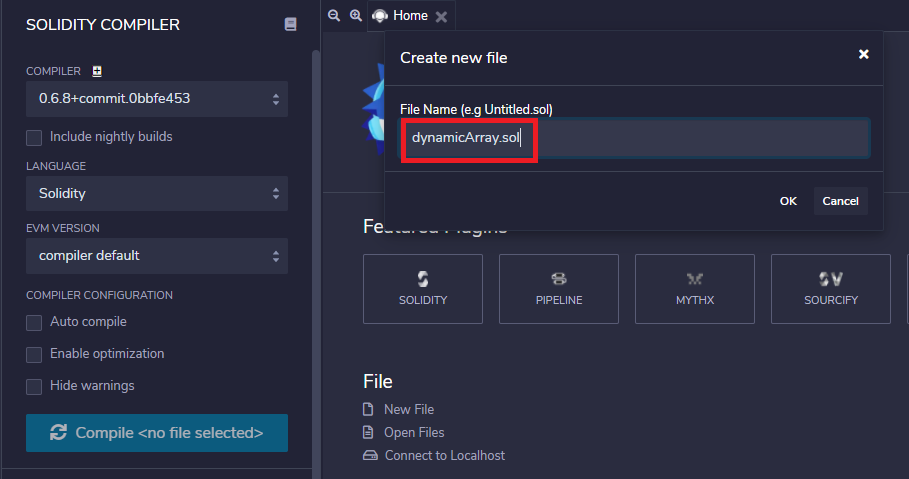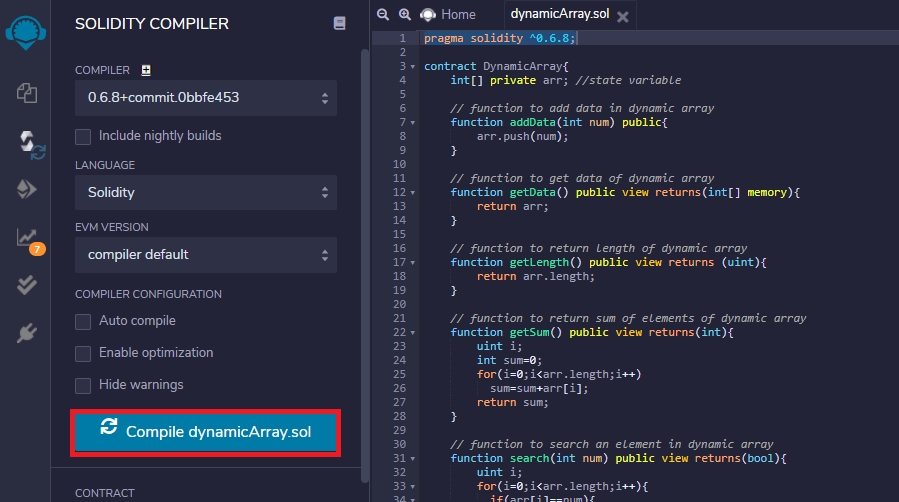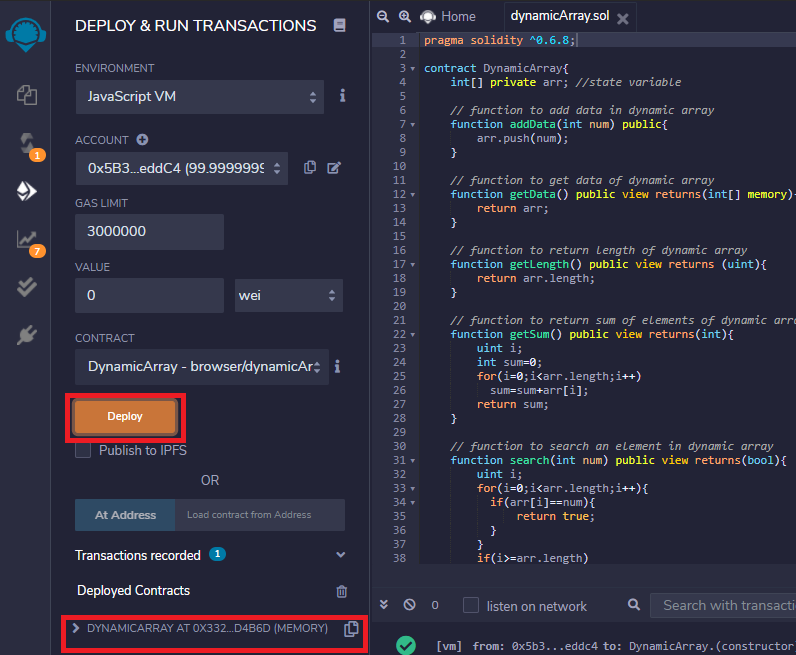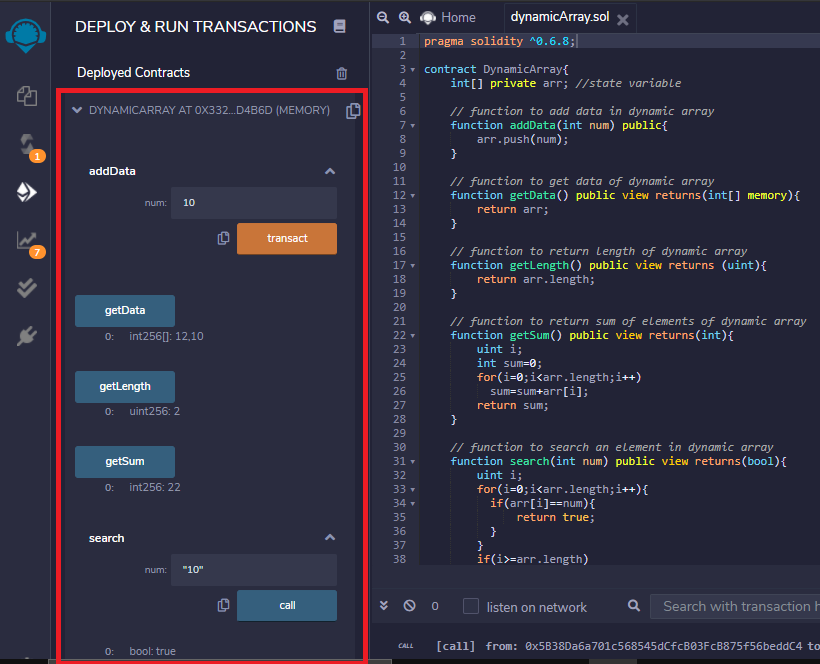# Dynamic Arrays and its Operations in Solidity

• Last Updated : 17 Nov, 2020

The Dynamic arrays are the arrays that are allocated memory at the runtime and the memory is allocated from the heap.

Syntax:

// declaration of dynamic array

int[] private arr;

How They Are Different From Fixed Size Arrays?

The fixed-size array has a fixed memory size whereas, in dynamic arrays, the size can be randomly updated during the run time which may be considered efficient with respect to the memory complexity of the code.

Problem: How to create a dynamic array in solidity and perform its associated operations?

Solution: In this article, we will create dynamic arrays in solidity language and will perform the following operations on it:

1. Add data in an array
2. Get data of an array
3. Get length of an array
4. Get sum of elements of an array
5. Search a particular element in an array

What is Solidity?

Solidity is a high-level language. The structure of smart contracts in solidity is very similar to the structure of classes in object-oriented languages. The solidity file has an extension .sol.

What are Smart Contracts?

Solidity’s code is encapsulated in contracts which means a contract in Solidity is a collection of code (its functions) and data (its state) that resides at a specific address on the Ethereum blockchain. A contract is a fundamental block of building an application on Ethereum.

Step 1: Open Remix-IDE.

Step 2: Select File Explorer from the left side icons and select Solidity in the environment. Click on the New option below the Solidity environment. Enter the file name as dynamicArray.sol and Click on the OK button.Step 3: Enter the following Solidity Code. Select the same solidity version as in your code.

## Solidity

 `// Solidity program to demonstrate``// the above approach``pragma solidity ^0.6.8;``contract` `DynamicArray{``   ` `// Declaring state variable  ``int``[] ``private` `arr; ``     ` `// Function to add data ``// in dynamic array``function addData(``int` `num) ``public``{``  ``arr.push(num);``}``     ` `// Function to get data of``// dynamic array``function getData() ``public` `view returns(``int``[] memory)``{``  ``return` `arr;``}``     ` `// Function to return length ``// of dynamic array``function getLength() ``public` `view returns (uint)``{``  ``return` `arr.length;``}`` ` `// Function to return sum of ``// elements of dynamic array``function getSum() ``public` `view returns(``int``)``{``  ``uint i;``  ``int` `sum = 0;``   ` `  ``for``(i = 0; i < arr.length; i++)``    ``sum = sum + arr[i];``  ``return` `sum;``}``     ` `// Function to search an ``// element in dynamic array``function search(``int` `num) ``public` `view returns(``bool``)``{``  ``uint i;``   ` `  ``for``(i = 0; i < arr.length; i++)``  ``{``    ``if``(arr[i] == num)``    ``{``      ``return` `true``;``    ``}``  ``}``   ` `  ``if``(i >= arr.length)``    ``return` `false``;``}``}`

Step 4: Compile the file dynamicArray.sol from the Solidity Compiler tab.Step 5: Deploy the smart contract from the Deploy and Run Transaction tab.Step 6: Perform various operations on the array under the deployed contracts section.My Personal Notes arrow_drop_up